[Home|Historical|Relevance|Theory|Alkanes|Sub-alk|σ-π Conjugations|Cycloalkanes|Hetrings|Atrop|Prob]
Interactivity: JSmol(default)|Jmol (Java). Visibility: Show|Hide. Controls: , .Print|Help

## Substituted alkanes

In compounds of the generic structure, X-C-C-Y, where X and Y are both electronegative groups, the balance of conformational preference shifts from app to gaucheThis is now due to the orbital overlap (effect 1 in theory) starting to dominate over the other two controlling factors. The relative energies of the σ-orbitals and σ*-orbitals of C-X or C-Y bonds have the following order:

⇐ small σ-σ* gap | large σ-σ* gap ⇒
⇐ Large E(2) | Small E(2) ⇒
low energy acceptor: σ*C-F < σ*C-Cl < σ*C-O ≈ σ*C-+NMe3 < σ*C-C≡N < σ*C-N < σ*C-C < σ*C-H < σ*C-Si high energy acceptor:
high energy donor: σC-Sn > σC-Si > σC-H > σC-C > σC-N > σC-O > σC-Cl > σC-F low energy donor

Thus vertical E(2) values on the left hand side of this list, for which the σ-σ* energy gap is small, are large whilst those on the rhs are much weaker: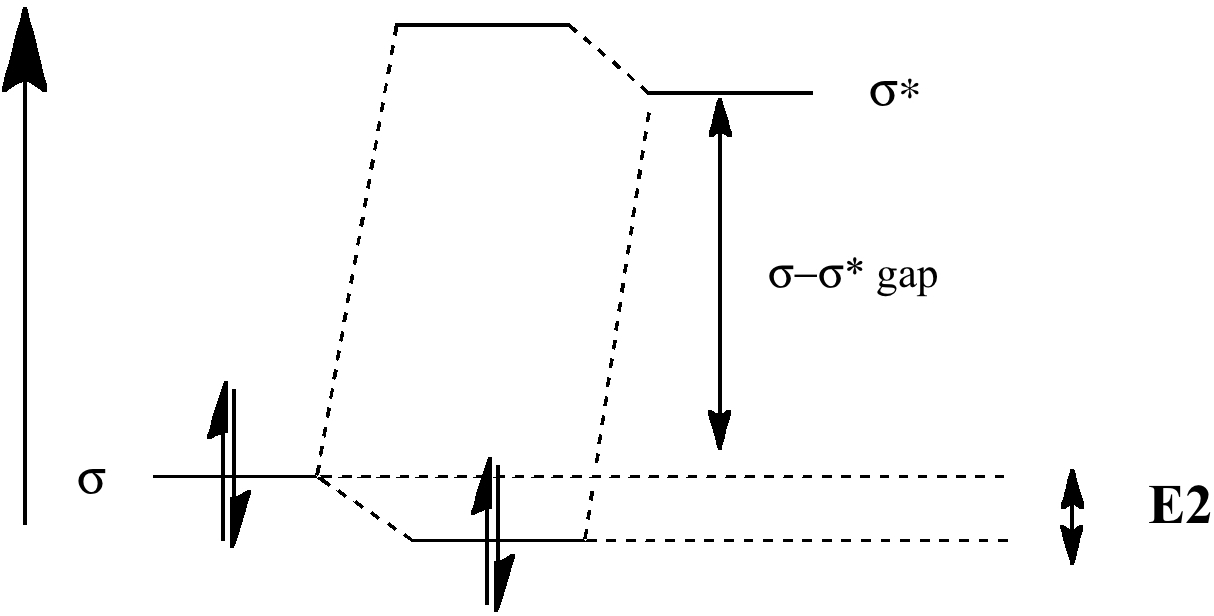### Examples: 1,2-Difluoroethane

gauche anti-periplanar (app)
1. two σC-H/σ*C-F interactions, E(2) = 4.9
2. two σC-H/σ*C-H, E(2) = 2.6
3. two σC-F/σ*C-H E(2)= 0.8
4. =16.6 kcal/mol in total
1. four σC-H/σ*C-H, E(2) = 2.5
2. two σC-F/σ*C-F, E(2) = 1.8
3. = 13.6 kcal/mol in total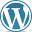The app is thus stabilized by ~3.0 kcal/mol less than the gauche.

### Examples: 1-trimethylsilyl-2-chloroethane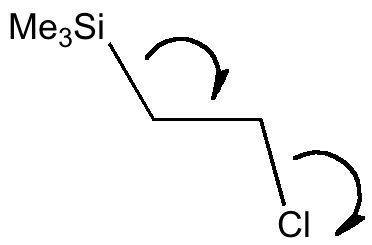An example involving C-Si as a better electron donor than C-H is shown below. This time the effect is to favour the anti-periplanar conformation, since the Si-C bond is a good donor, and C-Cl* is a good acceptor empty orbital (but not the other way around, σC-Cl/σ*C-Si, E(2) = 2.3 kcal/mol)

app conformation gauche conformation
σC-Si/σ*C-Cl, E(2) = 9.2
σC-H/σ*C-H, E(2) = 3.8
σC-H/σ*C-Cl, E(2) = 8.8 kcal/mol,
σC-Si/σ*C-H, E(2) = 3.3 kcal/molAs one moves down the periodic table, e.g. Sn-C is a better donor than Si-C. Conversely, Br-C is a much worse acceptor than F-C, and S-C is a worse acceptor than O-C. This effect also manifests in a search of the Cambridge crystal database for many combinations.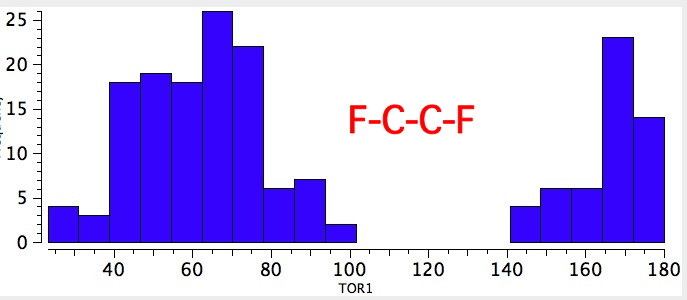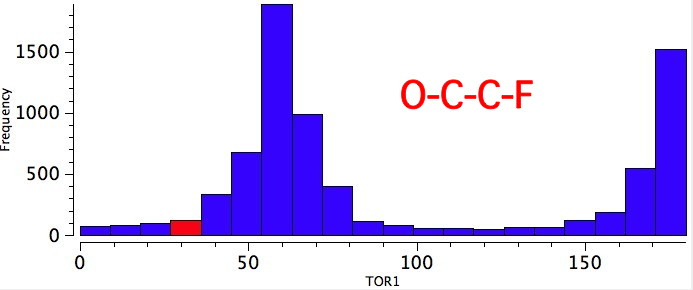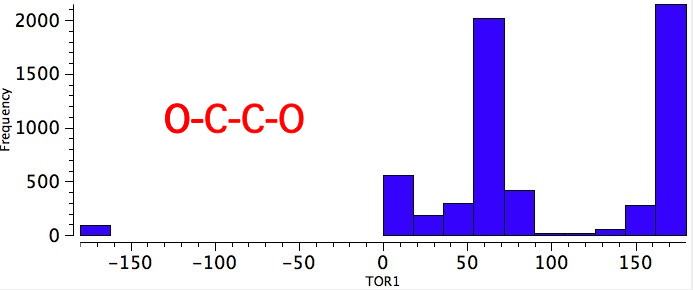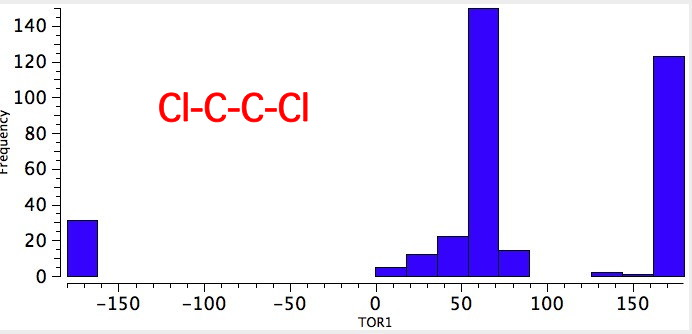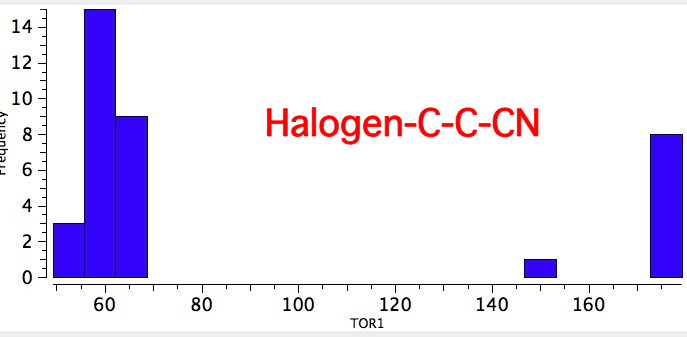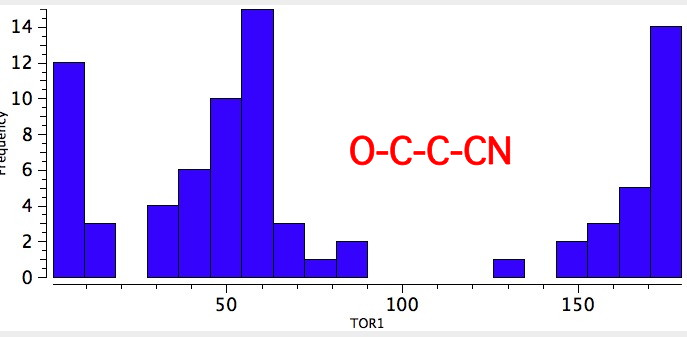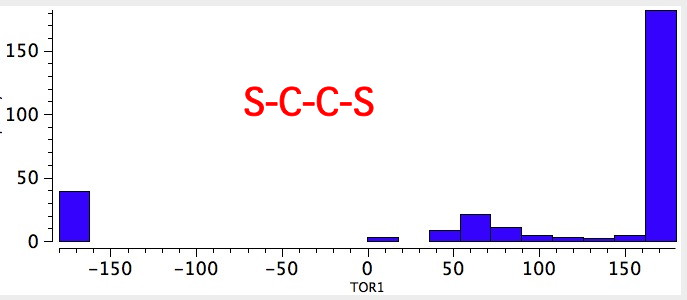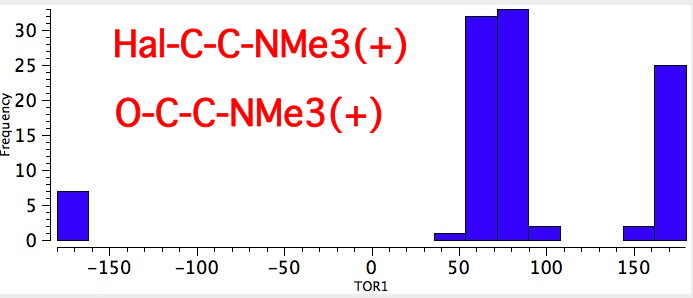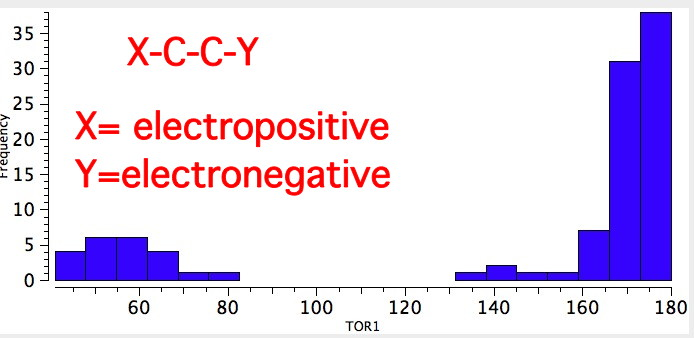### Example: Ethane-diol

In the case of 1,2-ethane-diol an intramolecular H-bond may also (weakly) stabilize the gauche form. A strong hydrogen bond does not form, because the O-H...O angle cannot adopt the preferred value of ~180°. Hydrogen bonds can also be thought of as arising from an interaction between a donor (Lp) and an acceptor H-X σ* interaction, and the overlap of that interaction is optimal for a co-linear angle. In that sense, the gauche effect and linear hydrogen bonds arise from exactly the same type of orbital interaction.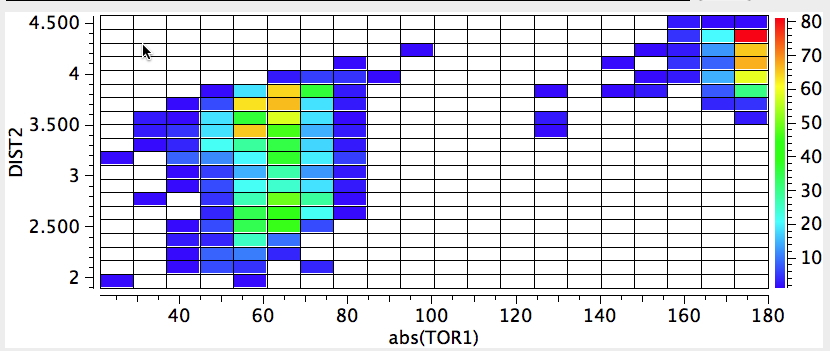### Example: An extreme case of the gauche effect. F-S-S-F

The record holder for the gauche effect is the molecule below:The rotational barrier for this molecule is > 25 kcal/mol, which gives it a reasonable kinetic life at room temperatures (t½ ~ 100 hours@298K). It thus crosses the boundary between a conformational isomer and a configurational isomer. Yes, it is also chiral (perhaps the smallest molecule capable of being so) and potentially resolvable into enantiomers.

## Summary of important concepts

1. In alkanes bearing strongly electronegative groups the gauche conformation is promoted because σC-H/σ*C-X interactions become dominant, and these occur as result of
• good orbital overlap in anti-periplanar orientations
• a low σC-H/σ*C-X energy gap
2. Hydrogen bonds are related to the gauche effect in terms of their orbital orientations and interactions.

© Henry S. Rzepa, 2010-2014. Hide|show Toolbar.